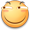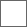• 156回复贴，共1

### 1 tree 3吨的拳力在一拳里有没有神级水平tree3》〉》〉》〉葛立恒数倍可观测宇宙TREE3吨力量，大约可以一瞬间打死琦玉TREE3次tree(3)和TREE(3)是不一样的，前者可能并不是极大(无法用指数塔表达)的数字i）a→b=a^b
ii）链A→1→链B=链A
iii）链→m'→n'=链→(链→m→n')→n

a→b→n=a↑…↑b /* n重箭头*/
3→3→64→2＜＜葛立恒数＜＜3→3→65→2＜＜＜3→3→3→3
＜＜＜＜＜3→3→3→3→3C(a)=a→[a]a
C(a，1)=a→[C(a)]a
C(a，b+1)=a→[C(a，b)]a
C(a，1，1)=C(a，C(a，a))
C(a，b+1，1)=C(a，C(a，b，1))
C(a，1，c+1)=C(a，C(a，a，c)，c)
C(a，b+1，c+1)=C(a，C(a，b，c+1)，c)
……（括号里的自变量个数可以无限增加）。。。。。。能秒葛立恒数个老师注意是大写小写是黑白画树，小了很多